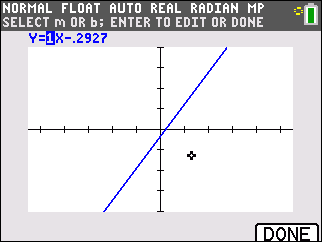# Knowledge Base

## Solution 34931: Using the Manual-Fit Function on the TI-84 Plus Family of Graphing Calculators.

### How can I use the Manual-Fit function on the TI-84 Plus family of graphing calculators?

Follow to the steps below to use the Manual-Fit function:

1) Press [MODE] and make sure FUNC is selected.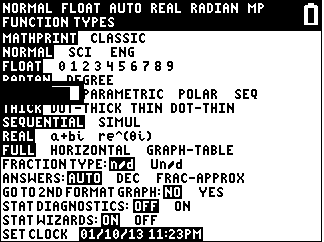2) Press [GRAPH].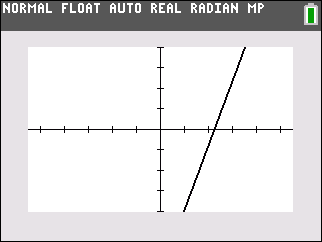3) Press [STAT] [►] and select D:Manual-Fit.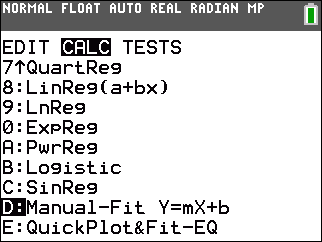4) From here, to store the equation, insert Y1 as the place to store the equation to. To do so, press [VARS] [►] and choose "Function".
5) Press [ENTER] on "Y1".
6) Press [▼] to "Calculate" and press [ENTER].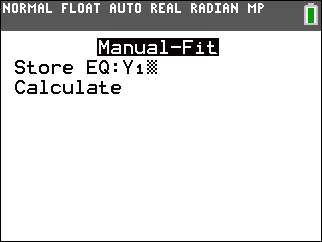7) Navigate to the first point and press [ENTER].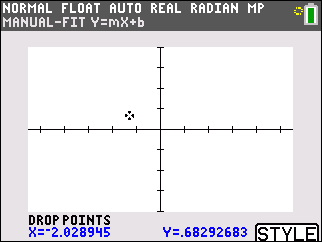8) Navigate to another point and press [ENTER] to mark a second point. A line containing the two points marked will automatically be drawn and the calculator will display the equation of the Manual-Fit Line in the form Y=mX+b, where m and b are the current values of the slope and y intercept (respectively). The current value of the first parameter (m) in the symbolic expression is highlighted.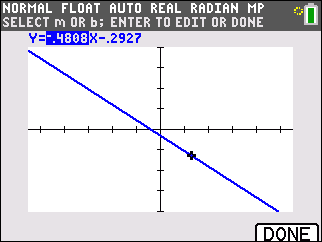9) To modify either m or b parameter select it and press [ENTER] for this example m is 1 and b is 2. Once the values are entered press [ENTER] again and the calculator will display the revised parameter value in the symbolic expression Y=mX+b, and refresh the graph with the updated Manual-Fit Line.